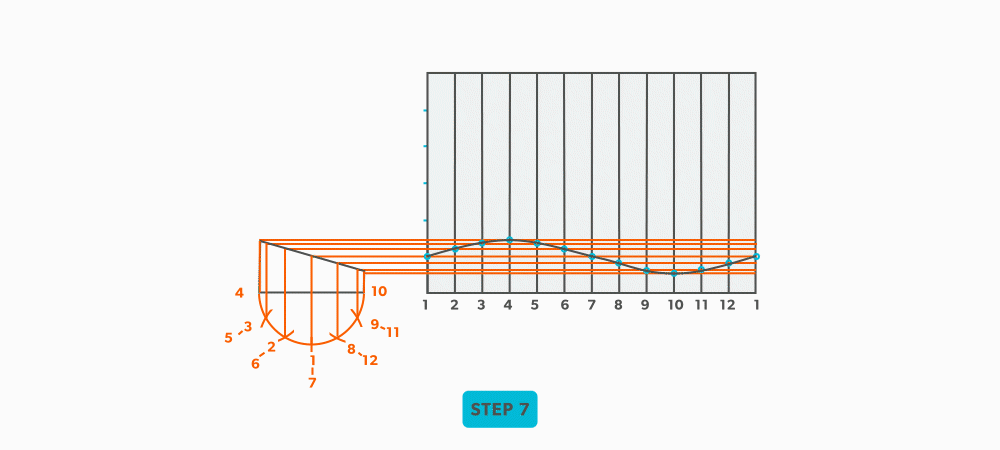Parallel Line Pattern Development

# 19 Round Elbow

For this example we will use a 6″ diameter-6″ throat radius-4pc-90°.
1. Use the (# of pcs × 2 − 2) to find the number of . For our example, it is 4 × 2 − 2 = 6. Each end piece is made up of 1 and each middle piece is made up of two gores.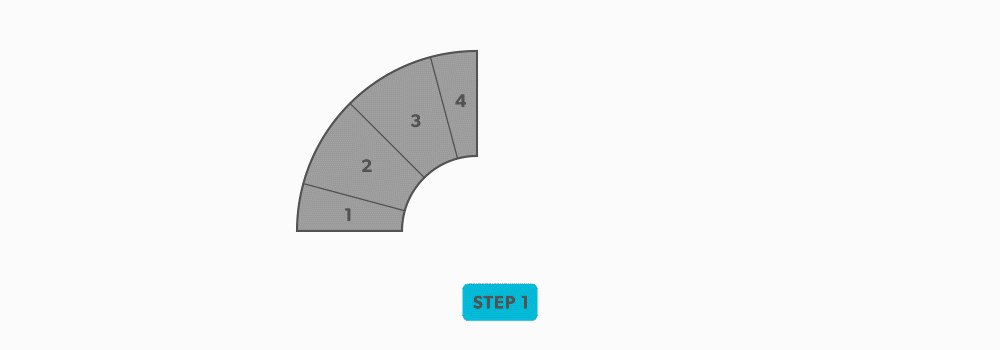2. Use the angle of the elbow divided by the number of gores to find the angle. In our case, 90° ÷ 6 = 15°.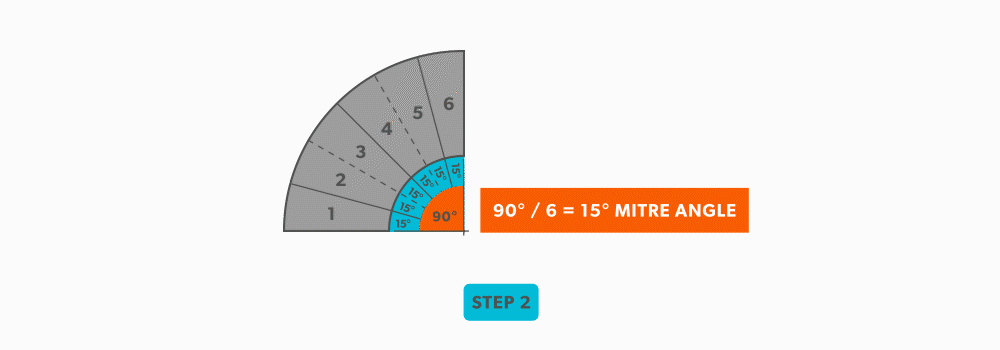3. Now that we know the miter angle is 15°, we can use an end gore and lay it out similar to a “Pipe on a Miter” (see Pipe on a Miter).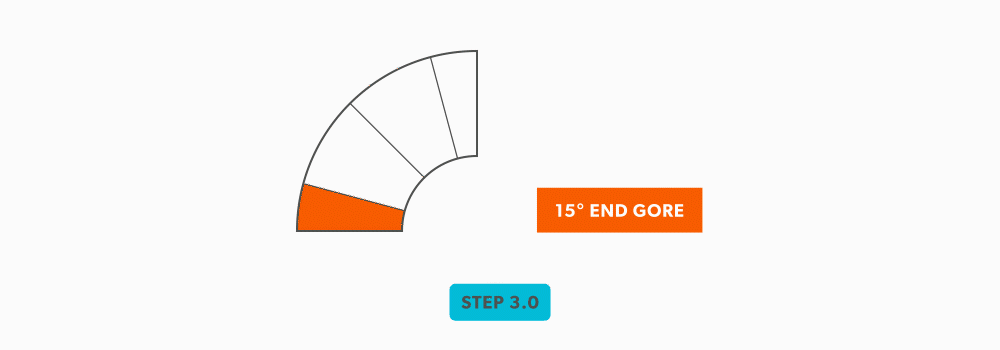1. Draw an , complete with the miter line.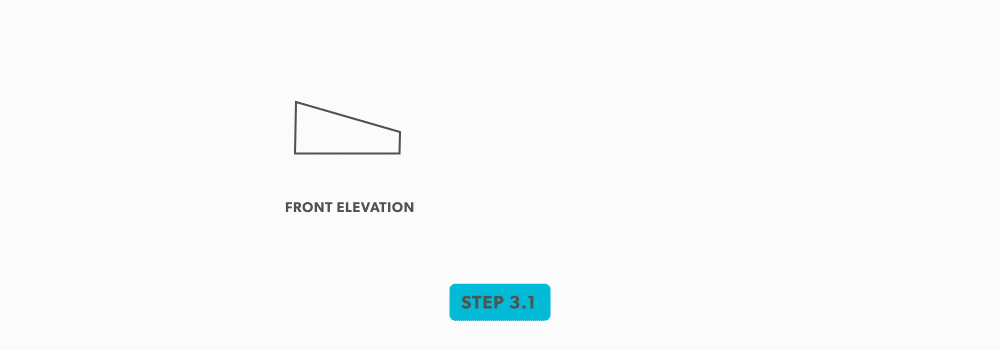2. Draw a profile below (see Divide a Circle Into 12 Equal Parts) and label it.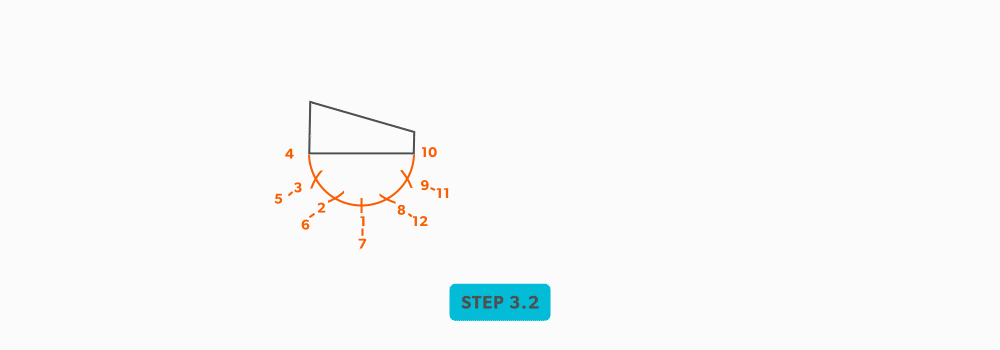3. Project the divisions up into the elevation view.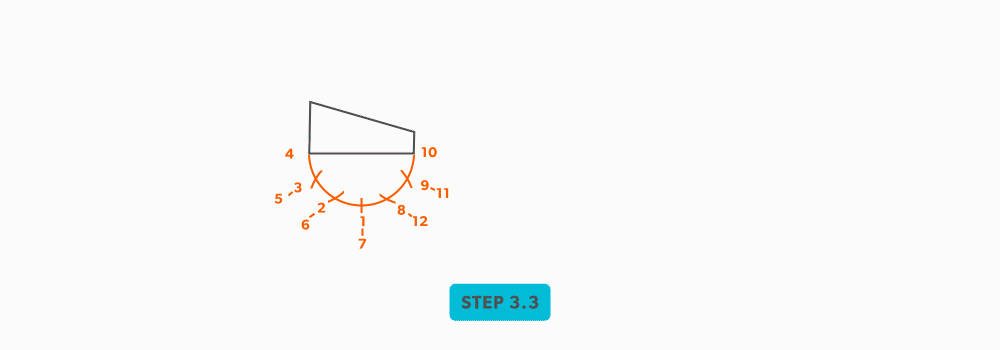4. Draw the (6 × Pi) directly to the right of the elevation view and divide it into 12 equal parts (see Divide a Line Using a Ruler on an Angle). Label it to match the elevation view and make sure to start the labeling on centre of the gore. Add any required seam allowances outside of the stretch-out.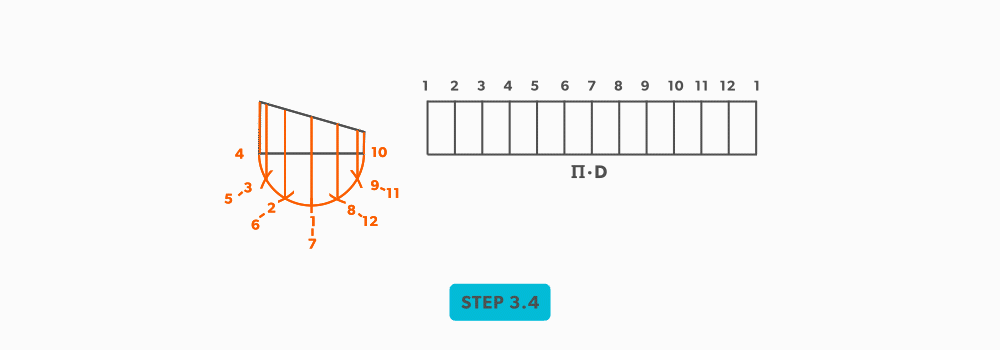5. At the points where the cross the miter line, project them into the stretch-out.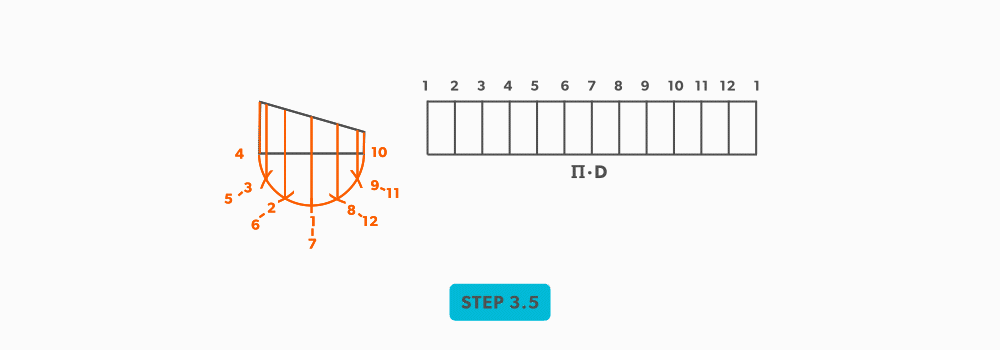6. Following the labeling, circle the intersection points on the stretch-out. Don’t put a dot over the points, but circle around them.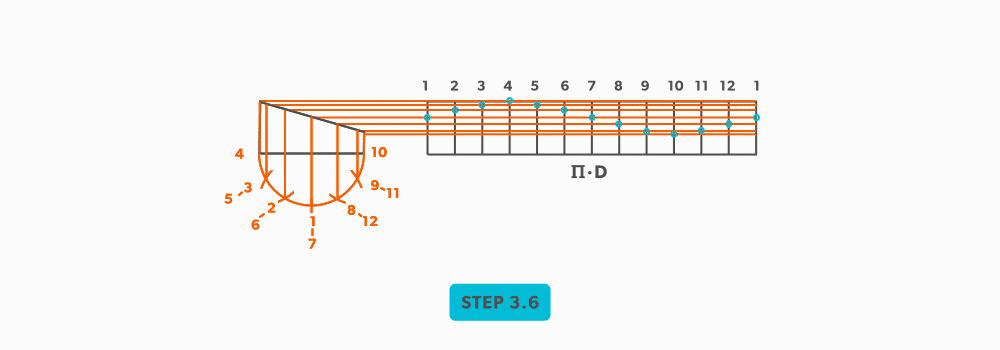7. Using a flexible curve, join the points to draw in the miter line, completing the .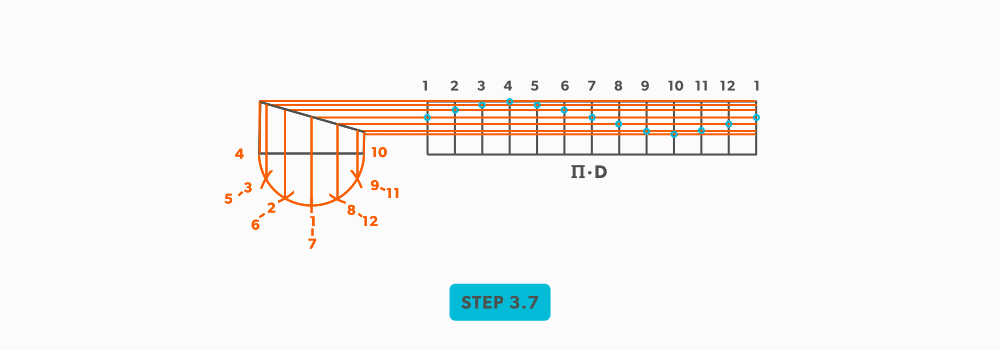4. We now need to finish the calculation for the blank size of the elbow. We already have the stretch-out, but we need the height. This is found by multiplying the seam height by the number of gores. For our example, simply measure the elevation view and find the height of the element line on centre of the gore. This should be 2 7/16″. 2 7/16″ × 6 =14 5/8″.
5. Now, cut out the blank size.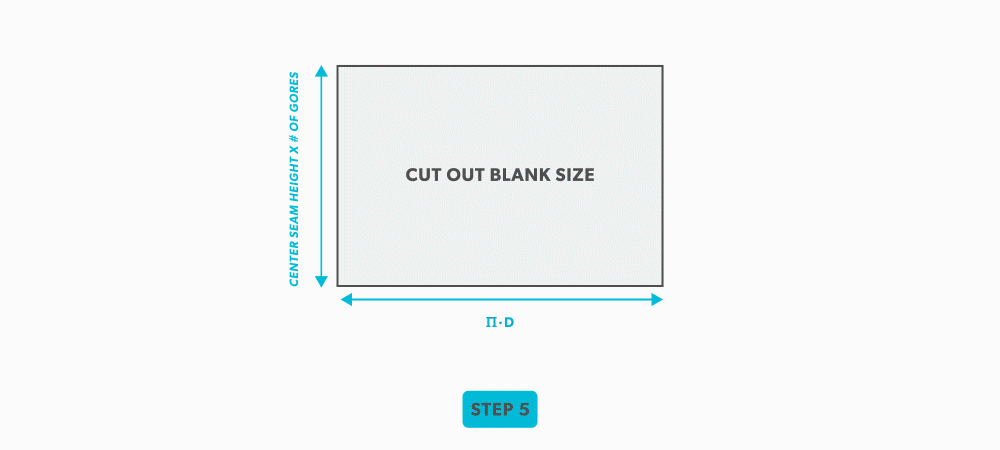6. Mark the seam height of each gore vertically on the stretch-out.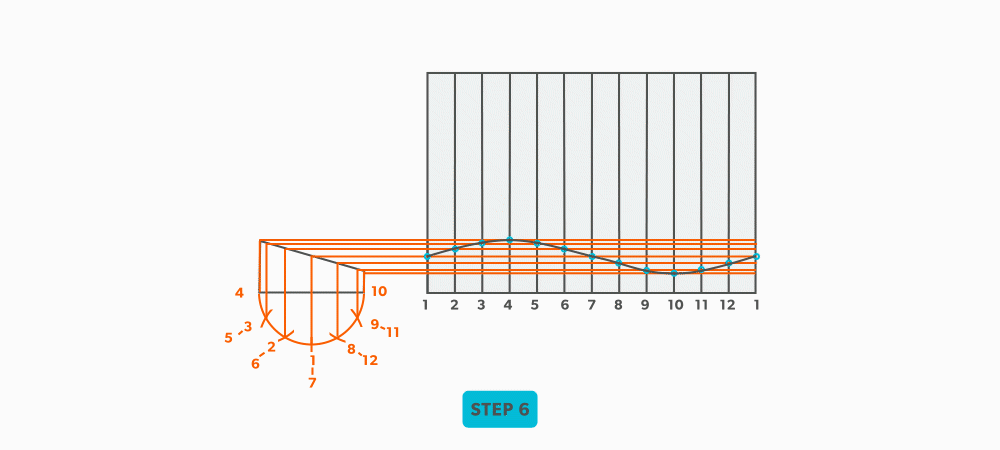7. To complete the elbow, trace or transfer the first gore pattern onto the blank and cut it out. Then, flip it and trace it for the rest of the gores. Do not flip left to right, only up and down and remember that you must leave 2 seam heights (2 gores) for the middle pieces. This will allow the seams to be orientated on opposing sides and produce the “fish” pattern.